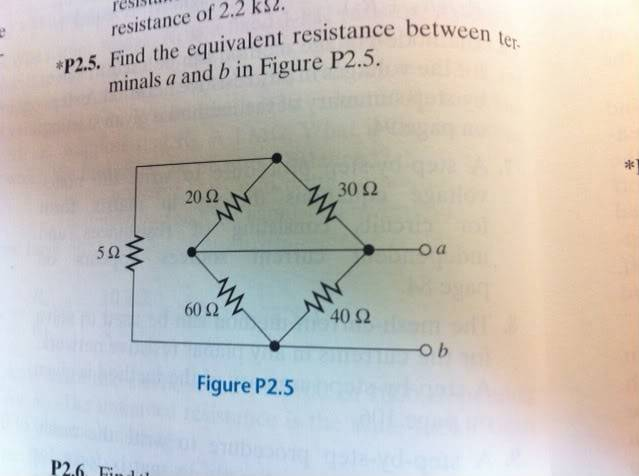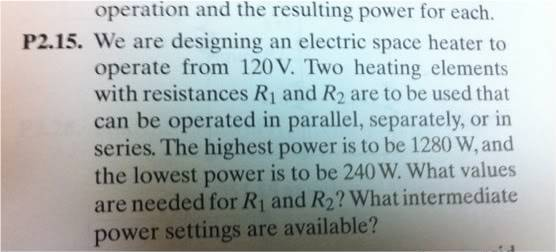# Basic Electrical Questions

dillonmhudson

## Homework Statement

Two questions:
1.2.## Homework Equations

P(power)=V(voltage)*I(current)
P=v^2/R
1/((1/R_1)+(1/R_2)+(1/R_n))

## The Attempt at a Solution1. I have simplified the bottom two resistors (60$$\Omega$$, 40$$\Omega$$) into one resistor using the equation 1/((1/R_1)+(1/R_2)+(1/R_n)) and have a single resistor of 24$$\Omega$$. Then I do the same with the top two (20$$\Omega$$, 30$$\Omega$$) and get a result of 12$$\Omega$$. I then combine the 12$$\Omega$$ and the 5$$\Omega$$ on the left by simple addition and get 17$$\Omega$$. Then, I combine the 17$$\Omega$$ in parallel with the 24$$\Omega$$ and get a simplified result of approx 9.95$$\Omega$$. I don't believe this to be right, however. My prof states that the answer is 9.6 - so I'm close, but no cigar.2. I use the equation P(power)=V(voltage)*I(current)
I substitute I for V/R (ohm's law) and get P=v^2/R. Using this equation I plug and chug 120V and either 1280W or 240W and get 11.25$$\Omega$$, and 60$$\Omega$$, respectively. I don't know what to do next however.
I tried the following:
1/((1/60$$\Omega$$)+(1/x))=11.25$$\Omega$$
Then using my TI-89 I solve for x and get a result of 13.85$$\Omega$$. I can therefore put 60$$\Omega$$ and 13.85$$\Omega$$ into parallel with a voltage source and get a power of either 1280W or 240W, correct?
The answers for 2 are 15$$\Omega$$ and 45$$\Omega$$ - wrong again.

Thanks for any help.

## Answers and Replies

Gold Member
For the second question you are on the right track with 11.25R and 60R, but you need to figure out what two resistances when put in parallel result in 11.25R and when put in series result in 60R.

Try turning that sentence into two separate equations with two separate unknowns, then solving simultaneously to get answers.

Then, don't forget to calculate each single resistive elements power (the intermediate power part of the question).

Last edited:
dillonmhudson
For the first question involving the resistors, all your initial calculations are correct. Why don't you believe them to be correct?

My prof got 9.6 and I got 9.95 for the R_eq. Really close and I don't know what could be the .3 difference. Thanks for the help, I guess I'll just keep it and argue it if it's wrong.

As for the second one, I'll take another shot at it.

Thanks!

Gold Member
I'd keep your result and ask to see his working to clarify where 'you' went wrong.

Working backwards, 40R||60R = 24R. If 24R||x = 9.6R, then x = 16R. If 5R + y = 16R, then y = 11R, but 20R||30R = 12R, so perhaps he just slipped on the calculator and put in 11R instead of 12R when he was doing that step?

I'm curious!

dillonmhudson
Hmmm perhaps. I'll certainly find out.

P.s. I have a friends solutions from last semester that I use as a guide (not very helpful as you can tell) and I saw he also had "||" written down in some places - what the heck does that mean?

Thanks!

Gold Member
Its a shorthand version of parallel.

Also useful is when only two resistances are in parallel, you can figure out their equivalent resistance by the formula (x * y) / (x + y) = R ... which is just R = 1 / (1 / x + 1 / y) rearranged (try it some time, its fast and handy to know).

i.e. 60R||40R = (60 * 40) / (60 + 40) = 2400 / 100 = 24R

dillonmhudson
That's a slick trick! By the way I got the second problem - thanks for the help!
I'll try and remember to post back if I ever get the first problem clarified.

dillonmhudson
Ok so for question one I found out why I was getting R_eq = 9.9 instead of 9.6 and I've found it is because I have the international edition of the book and the resistor on the left is 4$$Omega$$ instead of 5$$Omega$$ - sneaky.

Last edited: